Courses

# Ex 7.5 NCERT Solutions- Triangles Class 9 Notes | EduRev

## Mathematics (Maths) Class 9

Created by: Full Circle

## Class 9 : Ex 7.5 NCERT Solutions- Triangles Class 9 Notes | EduRev

The document Ex 7.5 NCERT Solutions- Triangles Class 9 Notes | EduRev is a part of the Class 9 Course Mathematics (Maths) Class 9.
All you need of Class 9 at this link: Class 9

Question 1. ABC is a triangle. Locate a point in the interior of ΔABC which is equidistant from all the vertices of ΔABC.
Soultion:
Let us consider a ΔABC.
Draw ‘l’ the perpendicular bisector of AB.
Draw ‘m’ the perpendicular bisector of BC.
Let the two perpendicular bisectors ‘l’ and ‘m’ meet at O. ‘O’ is the required point which is equidistant from A, B and C.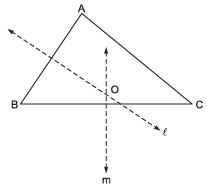Note: If we draw a circle with centre ‘O’ and radius OB or OC, then it will pass through A, B and C.

Question 2. In a triangle, locate a point in its interior which is equidistant from all the sides of the triangle.
Solution:
Let us consider a ΔABC.
Draw ‘l’ the bisector of ∠B.
Draw ‘m’ the bisector of ∠C.
Let the two bisectors l and m meet at O. Thus, ‘O’ is the required point which is equidistant from the sides of ΔABC.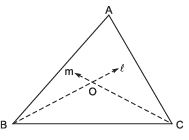required point which is equidistant from the sides of ΔABC.
Note: If we draw OM ⊥ BC and draw a circle with O as centre and OM as radius, then the circle will touch the sides of the triangle.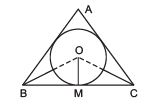Question 3. In a huge park, people are concentrated at three points (see figure): A : where there are different slides and swings form children,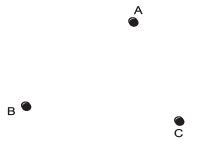B : near which a man-made lake is situated, C: which is near to a large parking and exit.
Where should an ice cream parlour be set up so that maximum number of persons can approach it?
Hint: The parlour should be equidistant from A, B and C.

Solution: Let us join A and B, and draw ‘l’ the perpendicular bisector of AB.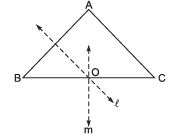Now, join B and C, and draw ‘m’ the perpendicular bisector of BC. Let the perpendicular bisectors ‘l’ and ‘m’ meet at ‘O’. The point ‘O’ is the required point where the ice cream parlour be set up.
Note: If we join ‘A’ and ‘C’, and draw the perpendicular bisectors, then it will also meet (or pass through) the point O.

Question 4. Complete the hexagonal and star shaped Rangolies [see Fig. (i) and (ii)] by filling them with as many equilateral triangles of side 1 cm as you can. Count the number of triangles in each case. Which has more triangles?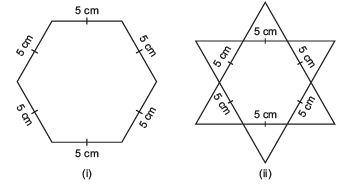Solution: It is an activity. We get the 150 equilateral triangles in the figure

(i) and 300 equilateral triangles in the figure

(ii). ∴ The figure

(ii) has more triangles.

,

,

,

,

,

,

,

,

,

,

,

,

,

,

,

,

,

,

,

,

,

;